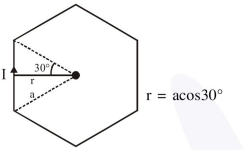# Magnitude of magnetic field (in SI units) at the centre of

Question:

Magnitude of magnetic field (in SI units) at the centre of a hexagonal shape coil of side $10 \mathrm{~cm}$, 50 turns and carrying current I (Ampere) in

units of $\frac{\mu_{0} I}{\pi}$ is :

1. $250 \sqrt{3}$

2. $5 \sqrt{3}$

3. $500 \sqrt{3}$

4. $50 \sqrt{3}$

Correct Option: , 3

Solution:$\mathrm{B}=\frac{6 \mu_{0} \mathrm{I}}{4 \pi \mathrm{a} \cos 30^{\circ}} \times 2 \sin 30^{\circ} \times 50$

$=\frac{\mu_{0} I}{\pi} \frac{150}{\sqrt{3} a}=\frac{50 \sqrt{3}}{0.1} \frac{\mu_{0} I}{\pi}$

$=500 \sqrt{3} \frac{\mu_{0} I}{\pi}$